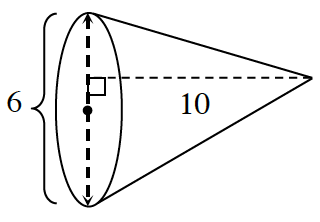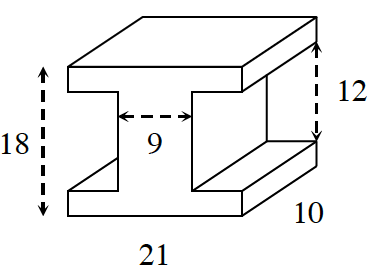### Home > CCG > Chapter 12 > Lesson 12.1.1 > Problem12-7

12-7.

Find the volume of each shape below. Assume that all corners in part (b) are right angles.

1. coneWhat is the formula for the volume of a cone?

$V= \frac{ 1 }{3 }(\pi)(3^2)(10) = 30 \pi = 94.2 \text{ un}^3$

1.Find the volume of an $18$ by $21$ by $10$ rectangular prism, then subtract the missing parts.

$2340$ un$^3$